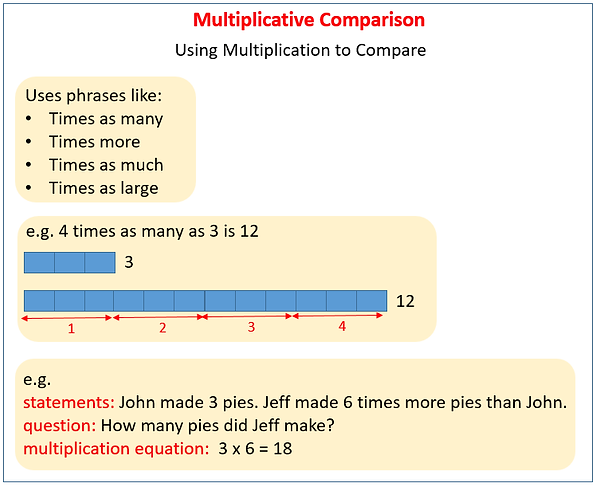top of page

MATH COMMON CORE STANDARDS

MULTIPLICATION AND DIVIDSION

OF WHOLE NUMBERS

More Practice4.NBT.6Find whole-number quotients and remainders with up to four-digit dividends and one-digit divisors, using strategies based on place value, the properties of operations, and/or the relationship between multiplication and division. Illustrate and explain the calculation by using equations, rectangular arrays, and/or area models.

4.NBT.5Multiply a whole number of up to four digits by a one-digit whole number, and multiply two two-digit numbers, using strategies based on place value and the properties of operations. Illustrate and explain the calculation by using equations, rectangular arrays, and/or area models.

4.OA.4

Find all factor pairs for a whole number in the range 1–100. Recognize that a whole number is a multiple of each of its factors. Determine whether a given whole number in the range 1–100 is a multiple of a given one-digit number. Determine whether a given whole number in the range 1–100 is prime or composite.

4.OA.5Generate a number or shape pattern that follows a given rule. Identify apparent features of the pattern that were not explicit in the rule itself. For example, given the rule "Add 3" and the starting number 1, generate terms in the resulting sequence and observe that the terms appear to alternate between odd and even numbers. Explain informally why the numbers will continue to alternate in this way.

## Learn the Divisibility Rules## Soft School Divisibility Rules Quiz## Learn About Prime and Composite Numbers

(Click on one of the links below to practice prime and composite numbers.)

bottom of page